Chapter 7 Class 9 Triangles
Concept wise

If two figures have the same shape & size,

they are congruent

For example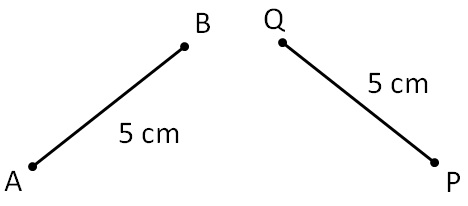Here,

AB & PQ have

• Same shape - both are lines
• Same size – both are 5 cm

So, they are congruent.

How to check if they are congruent?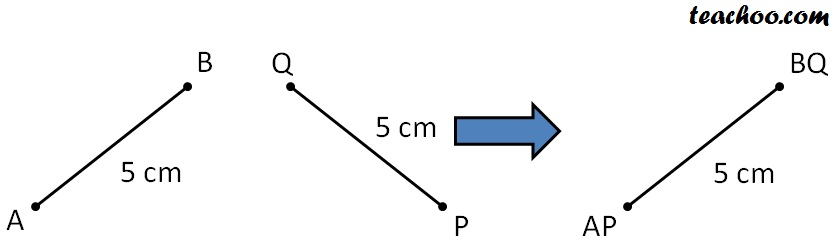We rotate PQ

& superimpose it an AB

Since, PQ comes AB exactly…

They are congruent

Some common shapes that are congruent are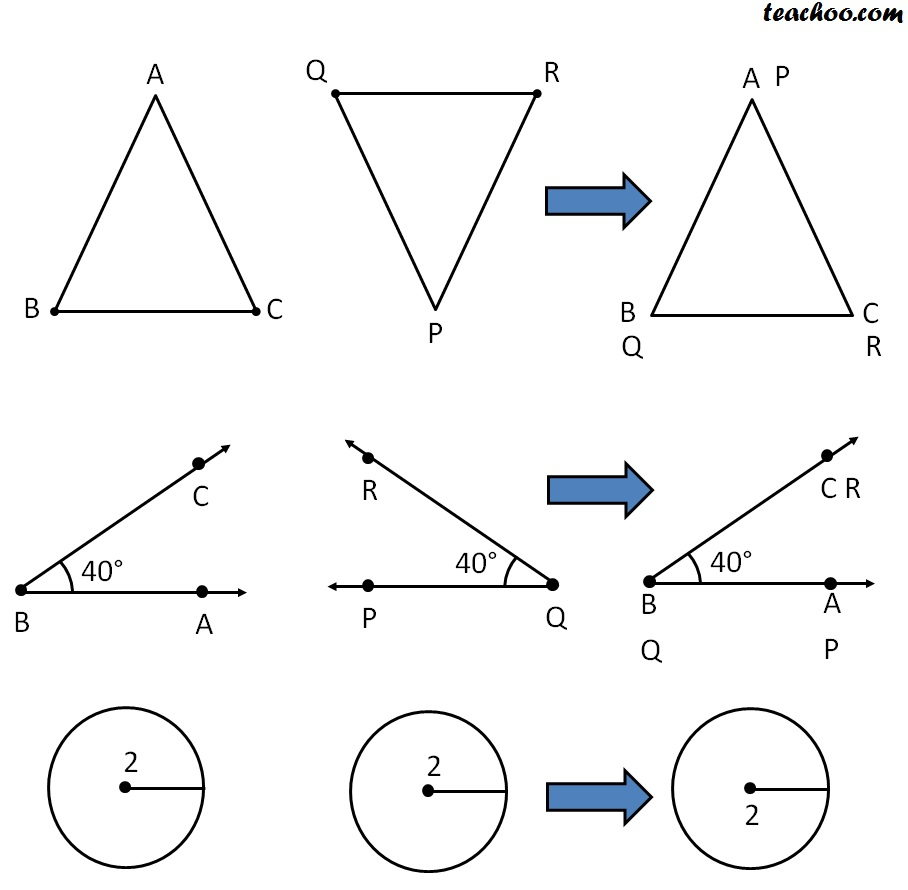Congruency Symbol

If two figures are congruent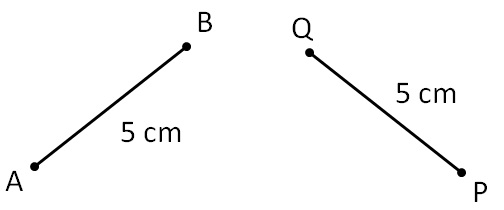We write

AB ≅ PQ

If two figures are not congruent like

We write,

XY ≇ CD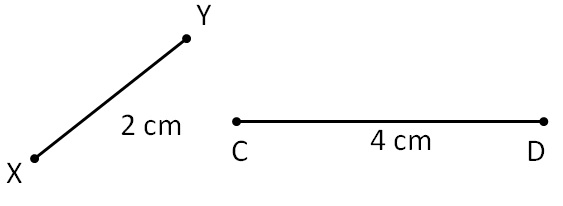Introducing your new favourite teacher - Teachoo Black, at only ₹83 per month